Home MonkeyNotes Printable Notes Digital Library Study Guides Study Smart Parents Tips College Planning Test Prep Fun Zone Help / FAQ How to Cite New Title Request

Example 41 The side of a cube are increasing at the rate of 0.5 cm/sec. At what rate its surface area and volume is increasing when the side is 10 cm long?

Solution : 1) The surface area (s) of a cube of side x is s = 6x2

By Differentiating w. r. to  t  , we get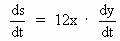where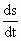= rate of change of surface area

and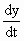= rate of change of the side of the cube.

Given that when x = 10 cm.= 0.5 cm /sec, then2) The volume (v) is given by v = x3

\\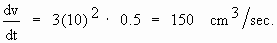Your browser does not support the IFRAME tag.

Example 42 The top of a ladder 20 units long is resting against a vertical wall and its foot on a level pavement, when the ladder beings to slide. When the foot of the ladder is 12 units from the wall, it is sliding away from the wall at the rate of 2 units / sec find the rate at which the top is sliding down.

Solution :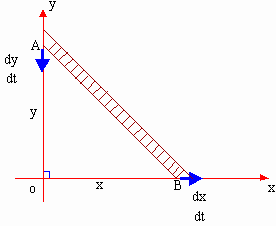Let AB = 20 units be the length of the ladder.

We have in right angled triangle AOB,

AB2 = AO2 +OB2

\ (20)2 = y2 + x2

i.e. x2 + y2 = 256

Also given that OB = x = 12 units

\ (12)2 + y2 = 400

\ y2 = 256

\ y = 16 units

Now differentiating w. r. to  t  , x2 + y2 = 400 we get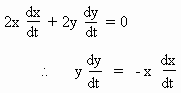Also.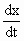= 2 units / sec = rate of sliding away from the wall.

\ 16= - 12(2)

\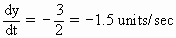\ The rate which the top is sliding down = 1.5 units/sec

Index

5.1 Tangent And Normal Lines
5.2 Angle Between Two Curves
5.3 Interpretation Of The Sign Of The Derivative
5.4 Locality Increasing Or Decreasing Functions 5.5 Critical Points
5.6 Turning Points
5.7 Extreme Value Theorem
5.8 The Mean-value Theorem
5.9 First Derivative Test For Local Extrema
5.10 Second Derivative Test For Local Extrema
5.11 Stationary Points
5.12 Concavity And Points Of Inflection
5.13 Rate Measure (distance, Velocity And Acceleration)
5.14 Related Rates
5.15 Differentials : Errors And ApproximationAll Contents Copyright © All rights reserved.
Further Distribution Is Strictly Prohibited.

620 PinkMonkey users are on the site and studying right now.